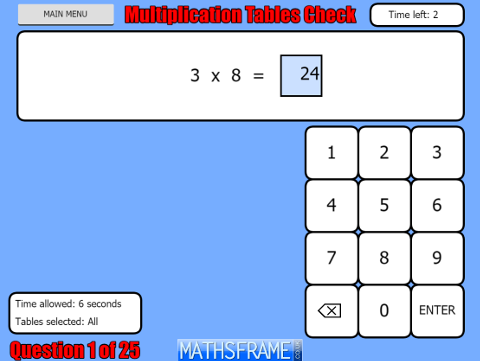Multiplication Tables Check

This activity exactly mirrors the 'Multiplication Tables Check' that will be given to children at the end of Year 4. They are tested on their multiplication tables up to 12 x 12. There are twenty-five questions and children have six seconds to answer each question and three seconds between questions. The questions are generated randomly using the same rules as the 'Multiplication Tables Check' (see below).

Results can be downloaded and printed at the end of the test.

A similar activity which tests recall of number bonds can be found here.